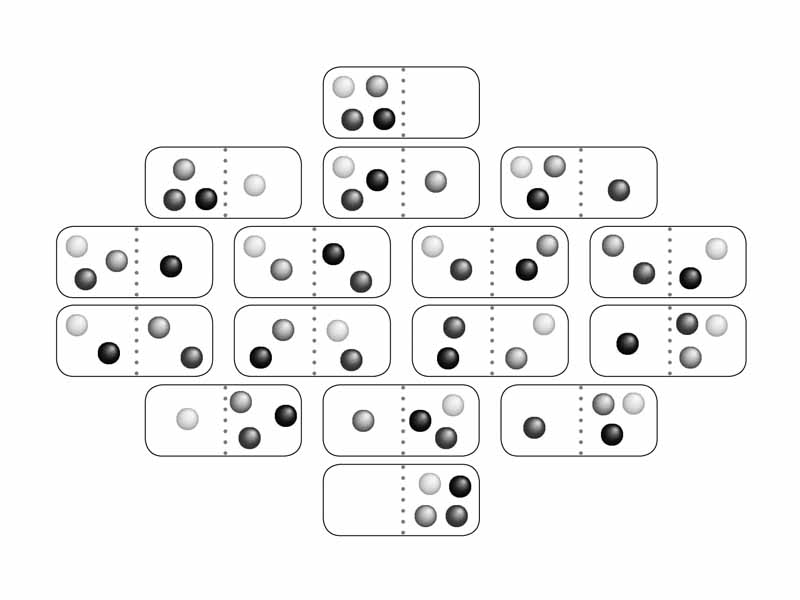A gas diffuses to fill a container. There are so many more microstates corresponding to that macrostate it is simple probability.

Let us work on our understanding of entropy from a somewhat more advanced perspective than you typically get in Chem 101. I believe a person needs to take one step further to get an intuitive grasp of these concepts, which are among the most important in science, especially as underpinnings to biochemistry, but keep in mind you aren't likely to be asked direct questions from this perspective. Okay. Here we go.

We are accustomed to thinking about a thermodynamic system in terms of its macroscopic properties (pressure, volume, temperature), and then from kinetic theory we can think terms of the individual states of motion of all of the particles of which it is composed. What do we mean by 'states of the particles'? A particle could be moving fast, slow, or somewhere in between. Think of all of the particles. Take a snap shot of the system and you have a statistical mechanical picture of one microstate. How many possible microstates are there? If only one particle that was moving fast is now slow, that is a different microstate. So the number of possible microstates is huge. That number is the system's multiplicity. Like quantized electron orbitals, possible states of molecular motion exist as a series of waveforms which in one dimension would be described like a standing wave on a fixed string. The entire series represents the probability distribution for the particles in a system. All of the possible combinations of individual particle states is the multiplicity of the system.

Okay. What we are trying to get at is the sense that each particle has a number of definite ways to be. The number of quantum states of a particle in an energy range for a gas depends on the space available and the temperature. So at a higher temperature, the gas particles have more states available, while a low temperature, less. For example, the reduced heat capacity of crystals at low temperature is evidence that quantum theory describes molecular motion (in this case vibrational) as a series of energy levels. At low temperatures, the amounts of energy transferred through molecular collisions are less than sufficient to raise atoms between vibrational states, so the heat capacity is close to zero.

The equation giving the fraction of gas molecules with velocities in a certain range is the Maxwell-Boltzmann distribution law. It gives the most probable way of distributing the total energy of the system among the molecules, the distribution with the maximum number of molecular states. Now here is the key concept. The greater the multiplicity of the system, the greater the entropy. A system with a great deal of multiplicity, has many ways to achieve the same macrostate, making that macrostate very likely. If a restraint prevents a system from achieving the state with the greatest multiplicity and this restraint is removed, the system will move spontaneously toward maximum multiplicity through simple probability. A gas diffuses across a container. There are just so many more microstates of the system involving the gas being spread out that it can shift between microstates nearly forever and it will not move back to occupy only one side. Increase in entropy occurs in expansion or mixing because of the increase in the multiplicity of quantum states.is that your intuitive feel be gathering steam. These concepts will help you understand a great deal about chemistry, although they are one step beyond premedical level.
